Clutch Prep is now a part of Pearson
Ch.9 - Bonding & Molecular StructureWorksheetSee all chapters

# Formal Charge

See all sections
Sections
Lewis Dot Symbols
Chemical Bonds
Dipole Moment
Octet Rule
Formal Charge
Lewis Dot Structures: Neutral Compounds
Lewis Dot Structures: Sigma & Pi Bonds
Lewis Dot Structures: Ions
Lewis Dot Structures: Exceptions
Lewis Dot Structures: Acids
Resonance Structures
Average Bond Order
Bond Energy
Coulomb's Law
Lattice Energy
Born Haber Cycle

Formal Charge is the charge given to an element within a molecule when assuming equal sharing of electrons between bonded elements.

###### Formal Charge

Concept #1: Formal Charge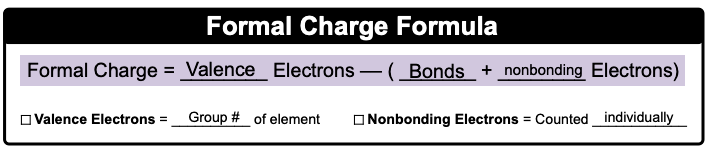Net Charge is the sum of individual formal charges within a compound.

Example #1: Determine the formal charge of the nitrogen atom found within the ammonia molecule, NH3.

Practice: Calculate the formal charges for each of the oxygen atoms within the nitrite ion, NO2.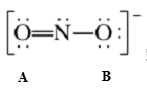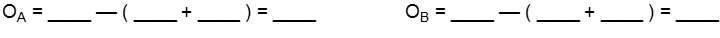Practice: Calculate the formal charge of the carbon atom within a carbon monoxide molecule.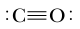a) –1

b) +2

c) 0

d) +

e) –2

Practice: Based on calculated formal charges, determine the overall charge (net charge) for the following compound.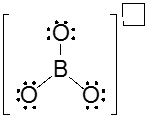a) –3

b) +4

c) +1

d) –1

e) +2

Practice: Which element within the thiocyanate ion possesses a negative charge?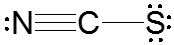a) C

b) S

c) N

d) All of them

e) None of them